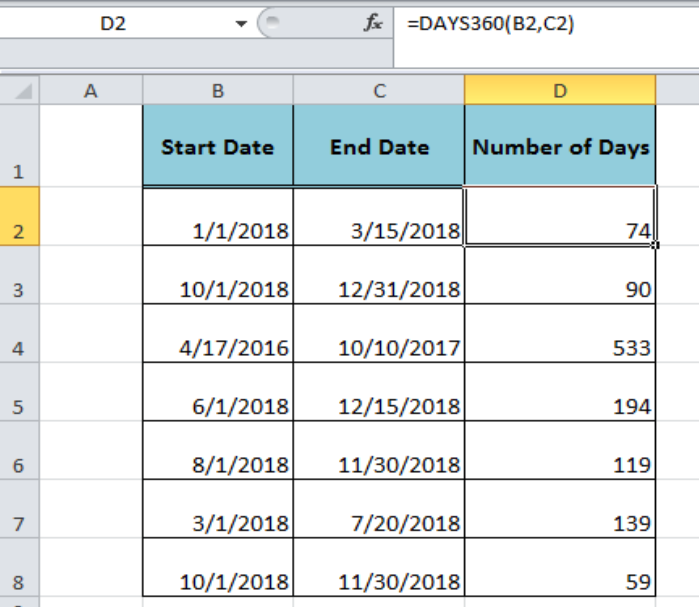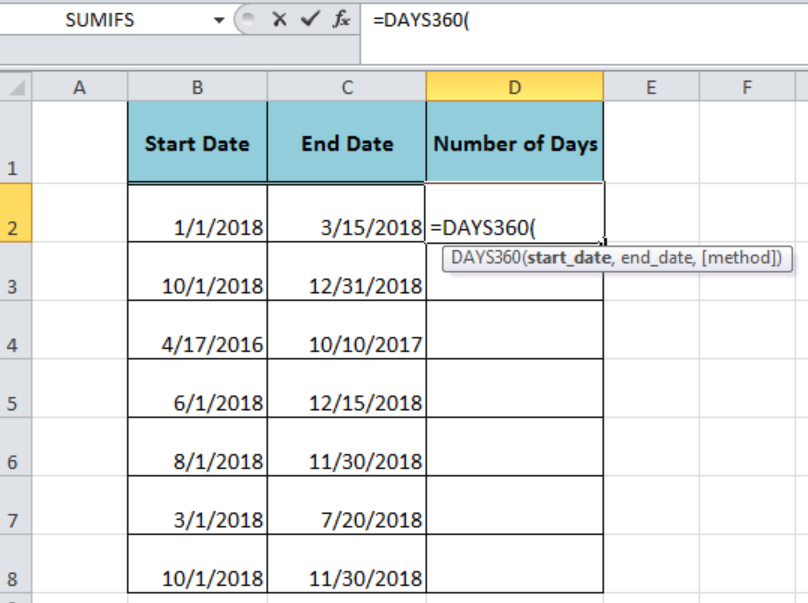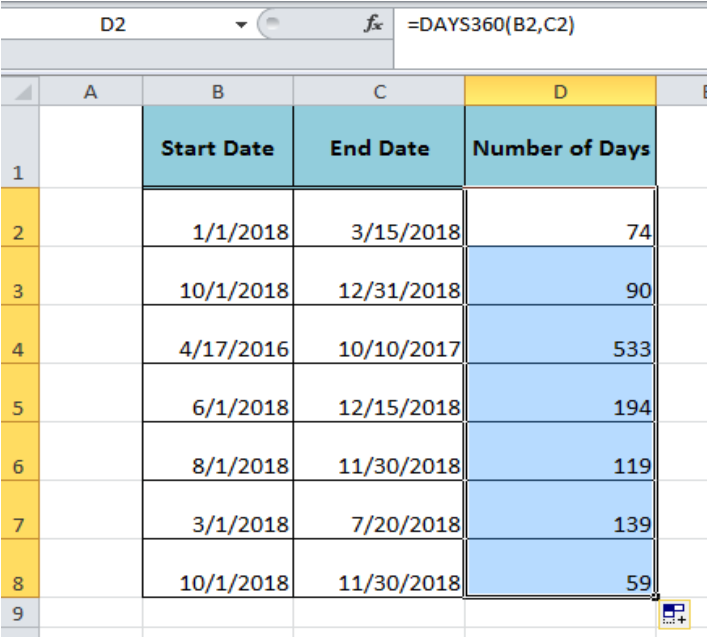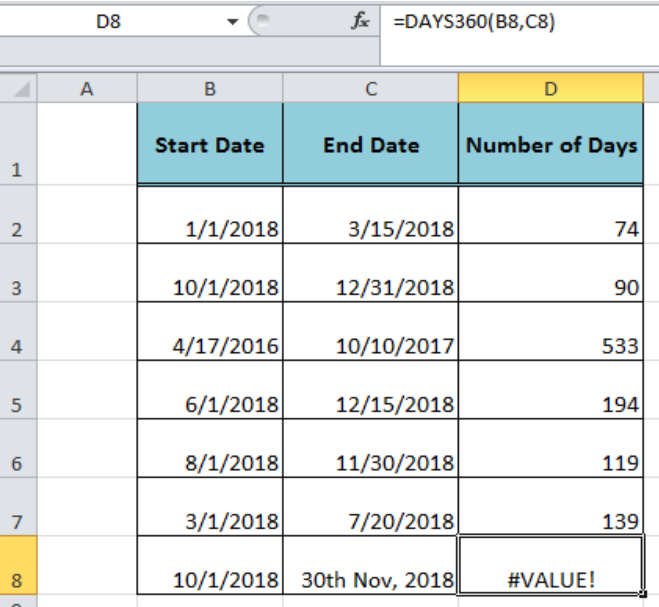Get instant live expert help with Excel or Google Sheets“My Excelchat expert helped me in less than 20 minutes, saving me what would have been 5 hours of work!”

#### Post your problem and you'll get expert help in seconds

Your message must be at least 40 characters
Our professional experts are available now. Your privacy is guaranteed.

# How to Use The Excel DAYS360 Function

Sometimes in accounting calculations, we need to calculate days between two dates based on a 360-days calendar year, twelve 30-day months. Therefore, the Excel DAYS360 function is used to return the number of days between two dates based on 360 days calendar yearFigure 1. Using the Excel DAYS360 Function

## Syntax

The syntax for the Excel DAYS360 function is;

`=DAYS360(start_date,end_date,[method])`

Where,

• start_date and end_date arguments are used to calculate the difference between two dates as the number of days.

• Method argument is optional and used to choose the US or European method. TRUE is selected for European and FALSE for the US method. The default method is the US method if omitted.Figure 2. The Syntax for the Excel DAYS360 Function

## Example

In our example, we have a table containing the start dates and the ending dates in a format supported by the Excel and we want to calculate the number of days between two dates as per the default US method. The Excel DAYS360 function calculates the number of days between two days in the following formula in cell D2;

`=DAYS360(B2,C2)`

Copy this formula down to other cells in column D.Figure 3. Calculating Days Between Dates With Excel DAYS360 Function

## Usage Notes for Excel DAYS360 Function

• It returns the #NUM! error if the start_date or end_date is a numeric value but not a valid date value.
• If we enter the start date or end date argument as a text value that cannot be represented as a date value then the function returns the #VALUE! error.Figure 4. An Error Value of Excel DAYS360 Function

## Instant Connection to an Expert through our Excelchat Service:

Most of the time, the problem you will need to solve will be more complex than a simple application of a formula or function. If you want to save hours of research and frustration, try our live Excelchat service! Our Excel Experts are available 24/7 to answer any Excel question you may have. We guarantee a connection within 30 seconds and a customized solution within 20 minutes.